# MATTER AND ATOMIC STRUCTURE matter anything that takes

• Slides: 25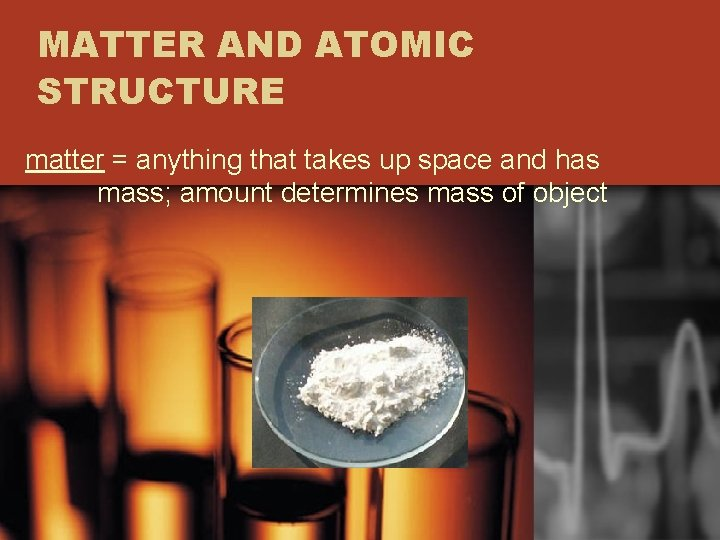MATTER AND ATOMIC STRUCTURE matter = anything that takes up space and has mass; amount determines mass of objectelements = cannot be broken down into simpler form by ordinary chemical means 1. forms of matter that contain only one type of atom 2. symbol of one or two letters represent each 3. periodic table = system for classifying elements 4. most abundant elements in universe = hydrogen & helium 5. silicon & oxygen make up 75% of earth’s crust; 92 natural elements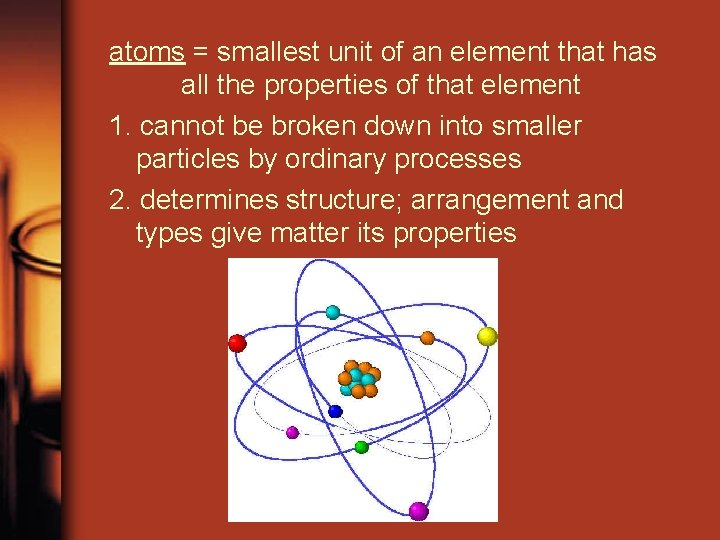atoms = smallest unit of an element that has all the properties of that element 1. cannot be broken down into smaller particles by ordinary processes 2. determines structure; arrangement and types give matter its propertiesATOMIC STRUCTURE 1. nucleus = core (center) a. protons = positive charge (p+) b. neutrons = neutral; no charge (n) c. has an overall positive charge due to protons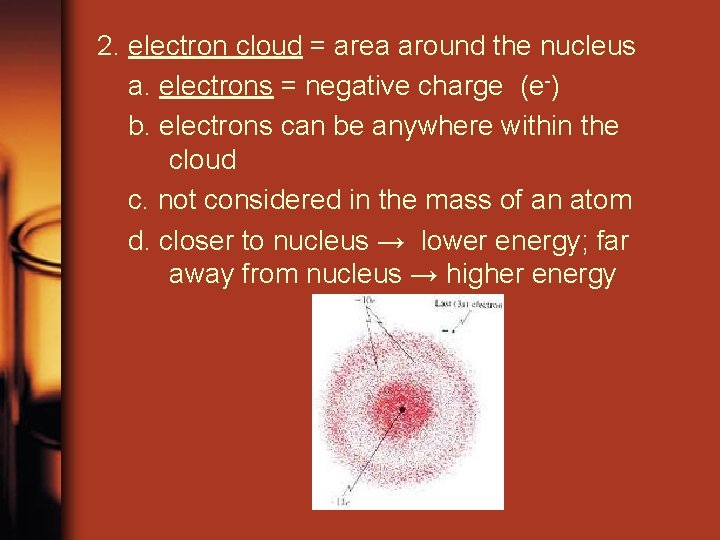2. electron cloud = area around the nucleus a. electrons = negative charge (e-) b. electrons can be anywhere within the cloud c. not considered in the mass of an atom d. closer to nucleus → lower energy; far away from nucleus → higher energyatomic number = number of protons in nucleus 1. also equals the number of electrons 2. unique to each element 3. when number of p+ equals the number of e- → atom is neutral 4. if number of p+ change → new element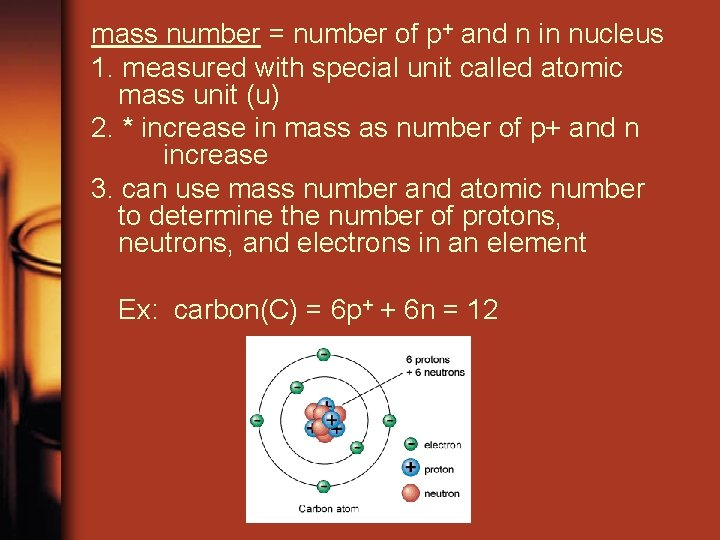mass number = number of p+ and n in nucleus 1. measured with special unit called atomic mass unit (u) 2. * increase in mass as number of p+ and n increase 3. can use mass number and atomic number to determine the number of protons, neutrons, and electrons in an element Ex: carbon(C) = 6 p+ + 6 n = 12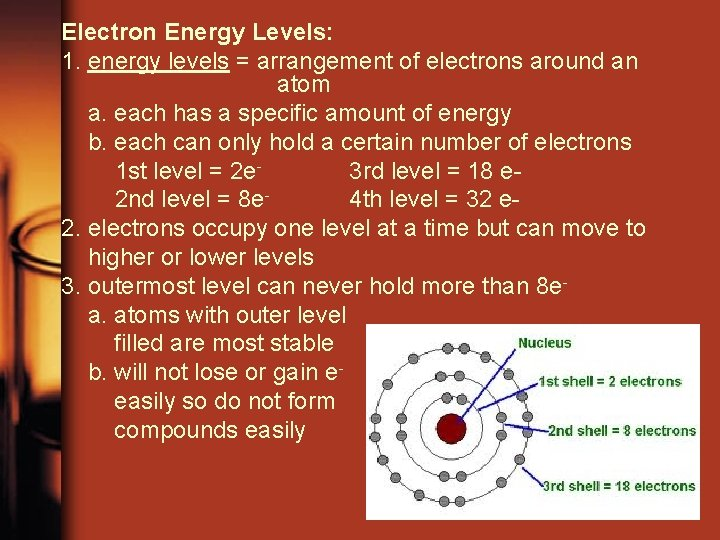Electron Energy Levels: 1. energy levels = arrangement of electrons around an atom a. each has a specific amount of energy b. each can only hold a certain number of electrons 1 st level = 2 e 3 rd level = 18 e 2 nd level = 8 e 4 th level = 32 e 2. electrons occupy one level at a time but can move to higher or lower levels 3. outermost level can never hold more than 8 ea. atoms with outer level filled are most stable b. will not lose or gain eeasily so do not form compounds easily4. valence electrons = outermost electrons; determine chemical behavior of element 5. atoms without outer level filled tend to exchange electrons a. metals = have only one, two, or three e- in outer level 1) good conductors of heat and electricity 2) malleable = can be hammered into thin sheets 3) ductile = can be drawn into wires 4) tend to give up electrons b. nonmetals = have four, five, or six electrons in outer level 1) tend to gain electrons when combine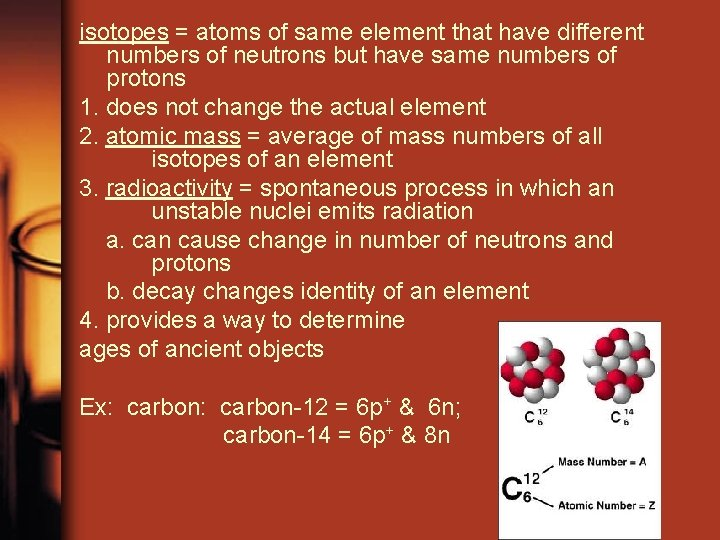isotopes = atoms of same element that have different numbers of neutrons but have same numbers of protons 1. does not change the actual element 2. atomic mass = average of mass numbers of all isotopes of an element 3. radioactivity = spontaneous process in which an unstable nuclei emits radiation a. can cause change in number of neutrons and protons b. decay changes identity of an element 4. provides a way to determine ages of ancient objects Ex: carbon-12 = 6 p+ & 6 n; carbon-14 = 6 p+ & 8 n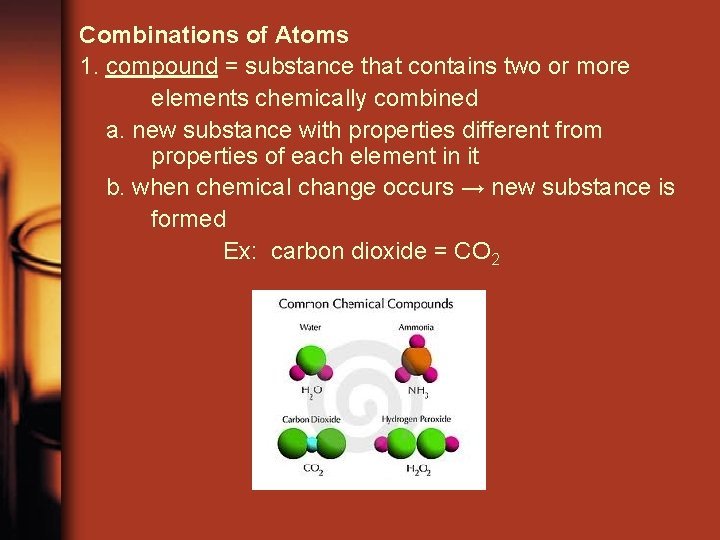Combinations of Atoms 1. compound = substance that contains two or more elements chemically combined a. new substance with properties different from properties of each element in it b. when chemical change occurs → new substance is formed Ex: carbon dioxide = CO 2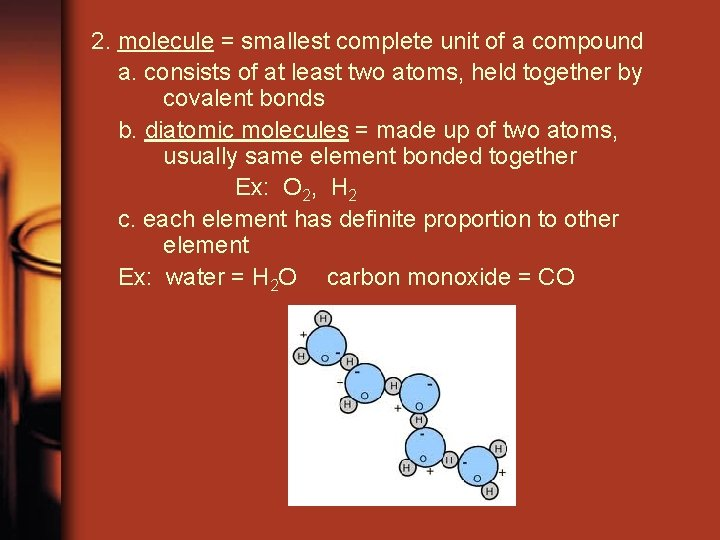2. molecule = smallest complete unit of a compound a. consists of at least two atoms, held together by covalent bonds b. diatomic molecules = made up of two atoms, usually same element bonded together Ex: O 2, H 2 c. each element has definite proportion to other element Ex: water = H 2 O carbon monoxide = CO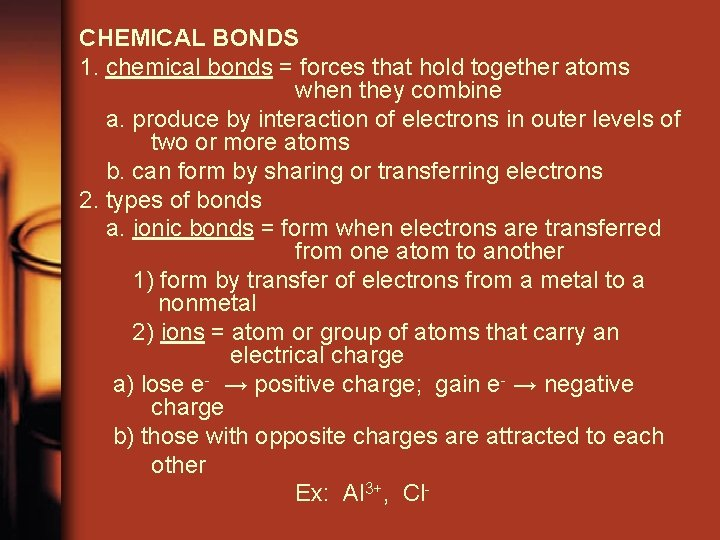CHEMICAL BONDS 1. chemical bonds = forces that hold together atoms when they combine a. produce by interaction of electrons in outer levels of two or more atoms b. can form by sharing or transferring electrons 2. types of bonds a. ionic bonds = form when electrons are transferred from one atom to another 1) form by transfer of electrons from a metal to a nonmetal 2) ions = atom or group of atoms that carry an electrical charge a) lose e- → positive charge; gain e- → negative charge b) those with opposite charges are attracted to each other Ex: Al 3+, Cl-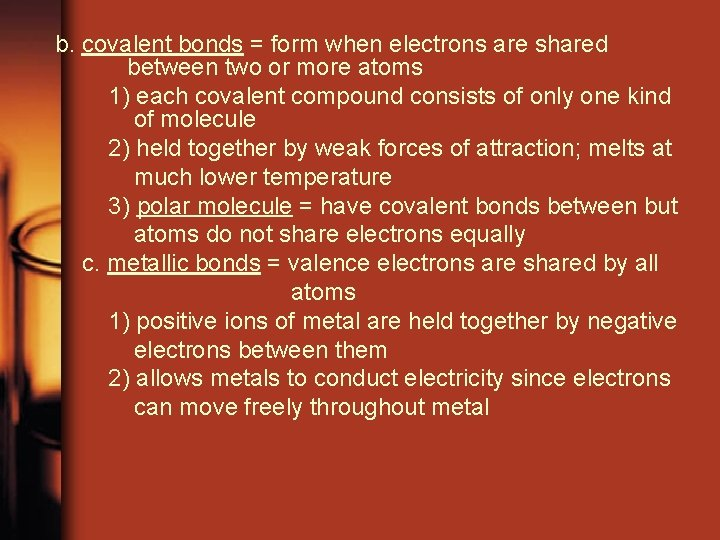b. covalent bonds = form when electrons are shared between two or more atoms 1) each covalent compound consists of only one kind of molecule 2) held together by weak forces of attraction; melts at much lower temperature 3) polar molecule = have covalent bonds between but atoms do not share electrons equally c. metallic bonds = valence electrons are shared by all atoms 1) positive ions of metal are held together by negative electrons between them 2) allows metals to conduct electricity since electrons can move freely throughout metal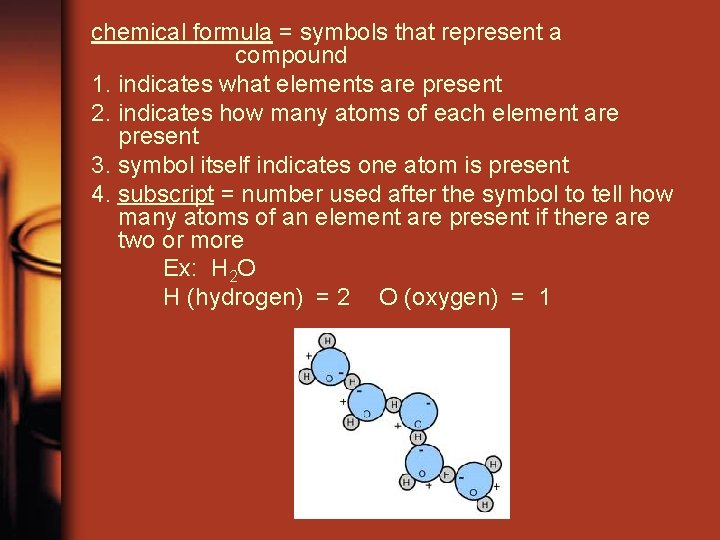chemical formula = symbols that represent a compound 1. indicates what elements are present 2. indicates how many atoms of each element are present 3. symbol itself indicates one atom is present 4. subscript = number used after the symbol to tell how many atoms of an element are present if there are two or more Ex: H 2 O H (hydrogen) = 2 O (oxygen) = 1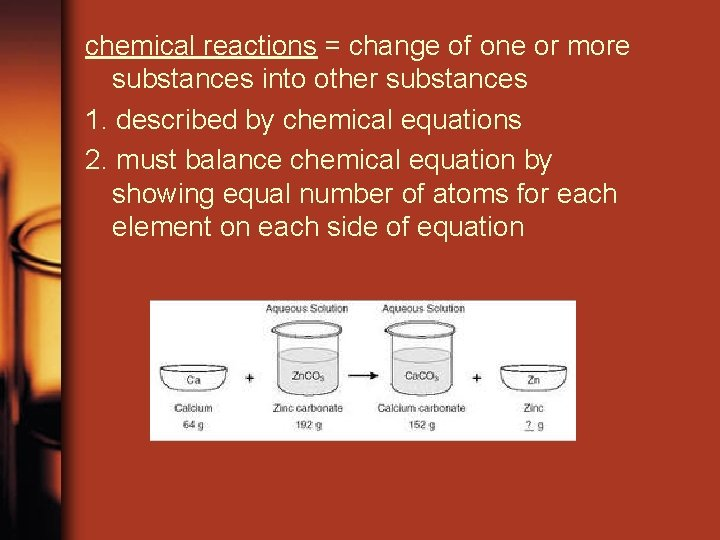chemical reactions = change of one or more substances into other substances 1. described by chemical equations 2. must balance chemical equation by showing equal number of atoms for each element on each side of equationMIXTURES: 1. mixture = contains two or more substances not chemically combined a. each substance retains its own properties b. components can be separated by physical means 2. heterogeneous mixture = components are easily recognizable Ex: soil 3. solution (homogeneous mixture) = one substance is uniformly dispersed in another substance a. one or more substances can/are dissolved in another substance b. parts: 1) solute = substance being dissolved (added) 2) solvent = substance the solute is dissolved in (put into) c. alloy = solution of two or more metals Ex: brass, bronze d. can be liquid, gas, or solid mixturesAcids & Bases: 1. acid = solution that has a substance that produces hydrogen ions (H+) a. most common acid in environment = carbonic acid b. major role in dissolution of minerals in soil 2. base = solution that has a substance that produces hydroxide ions (OH-) 3. p. H scale = measure hydrogen and hydroxide ions in solutions a. p. H = 7 (neutral) b. p. H > 7 (basic) Base c. p. H < 7 (acidic) [rainwater: p. H = 5. 0 to 5. 6 normally] AcidSTATES OF MATTER 1. solid = atoms/molecules in fixed position a. vibrate around a fixed point b. has definite shape, definite volume c. most have crystalline structure = particles arranged in regular geometric patterns 1) crystals for symmetrical solid objects with flat faces and straight edges between faces 2) well formed crystals are rare 3) polycrystalline substance = mass of intergrown crystals d. glass = molten material cooled so quickly atoms do not have time to arrange2. liquid = atoms/molecules free to move a. remain close as change positions b. has no definite shape, but has definite volume c. usually takes the shape of the container 3. gas = atoms/molecules move freely and independently a. particles are farther apart and move faster b. formless collection of particles that tends to expand in all directions at once c. has no definite shape, no definite volume 4. plasma = composed of ions and electrons a. higher energy than gas b. most matter in universe is in this state c. exist at very high temperatures Ex: sun and other stars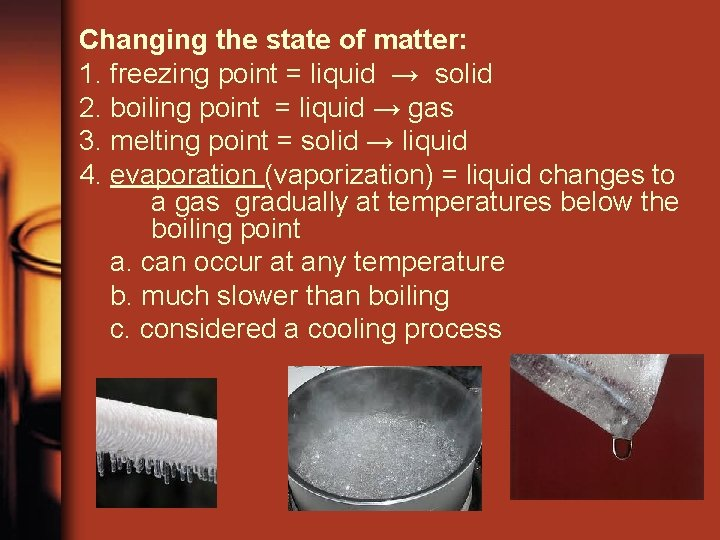Changing the state of matter: 1. freezing point = liquid → solid 2. boiling point = liquid → gas 3. melting point = solid → liquid 4. evaporation (vaporization) = liquid changes to a gas gradually at temperatures below the boiling point a. can occur at any temperature b. much slower than boiling c. considered a cooling process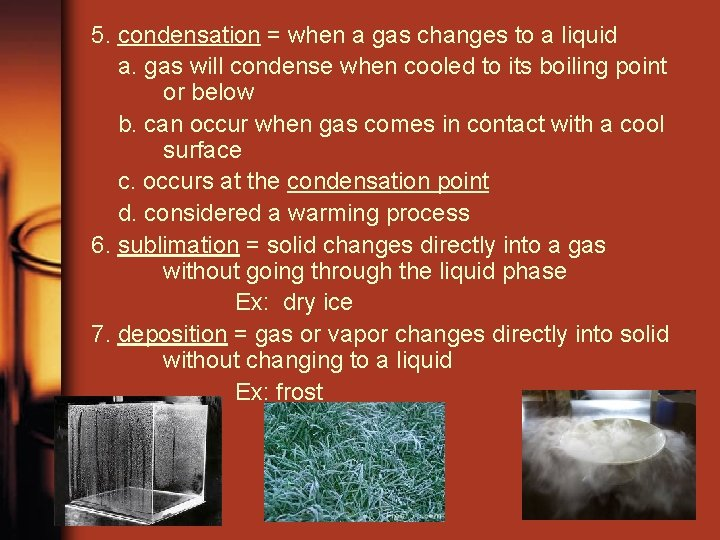5. condensation = when a gas changes to a liquid a. gas will condense when cooled to its boiling point or below b. can occur when gas comes in contact with a cool surface c. occurs at the condensation point d. considered a warming process 6. sublimation = solid changes directly into a gas without going through the liquid phase Ex: dry ice 7. deposition = gas or vapor changes directly into solid without changing to a liquid Ex: frostState of matter determined by: 1. attraction between atoms / molecules 2. rate of movement Can change state by changing: 1. temperature - different for different substances 2. increase or decrease the pressure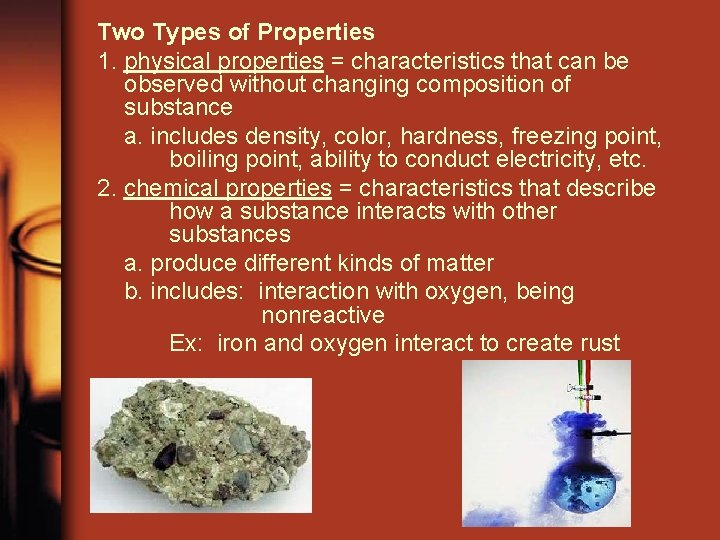Two Types of Properties 1. physical properties = characteristics that can be observed without changing composition of substance a. includes density, color, hardness, freezing point, boiling point, ability to conduct electricity, etc. 2. chemical properties = characteristics that describe how a substance interacts with other substances a. produce different kinds of matter b. includes: interaction with oxygen, being nonreactive Ex: iron and oxygen interact to create rust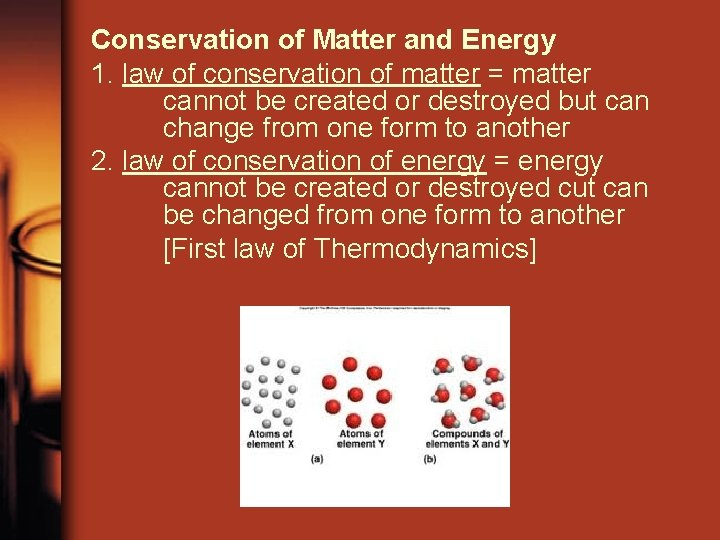Conservation of Matter and Energy 1. law of conservation of matter = matter cannot be created or destroyed but can change from one form to another 2. law of conservation of energy = energy cannot be created or destroyed cut can be changed from one form to another [First law of Thermodynamics]